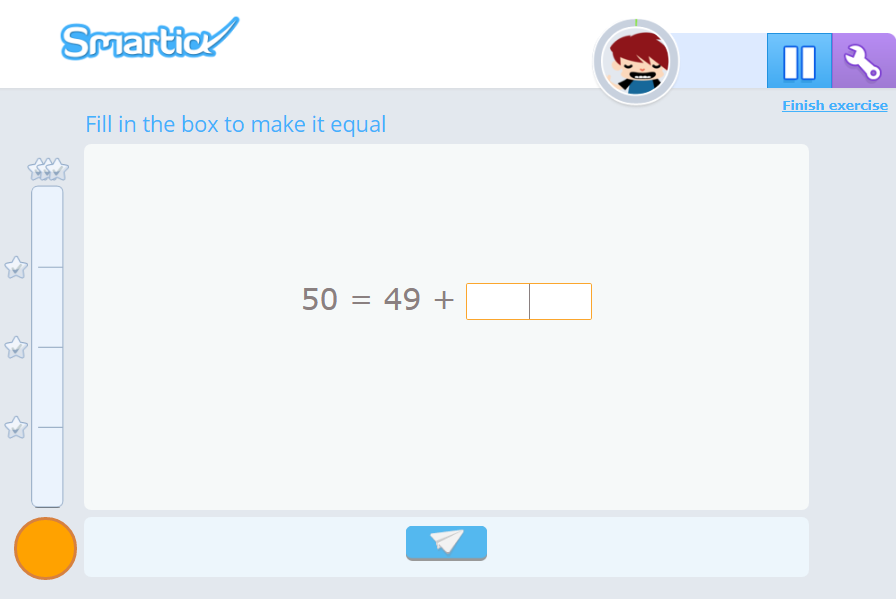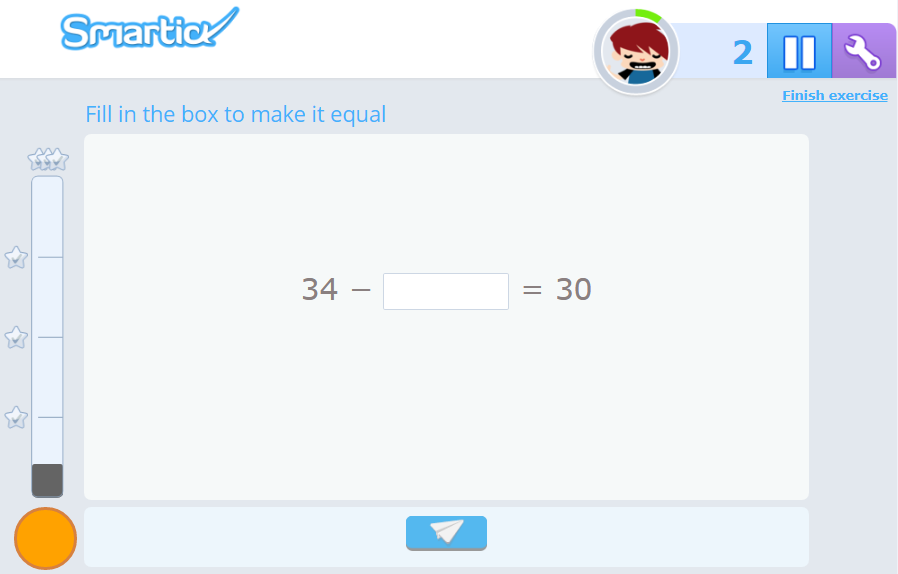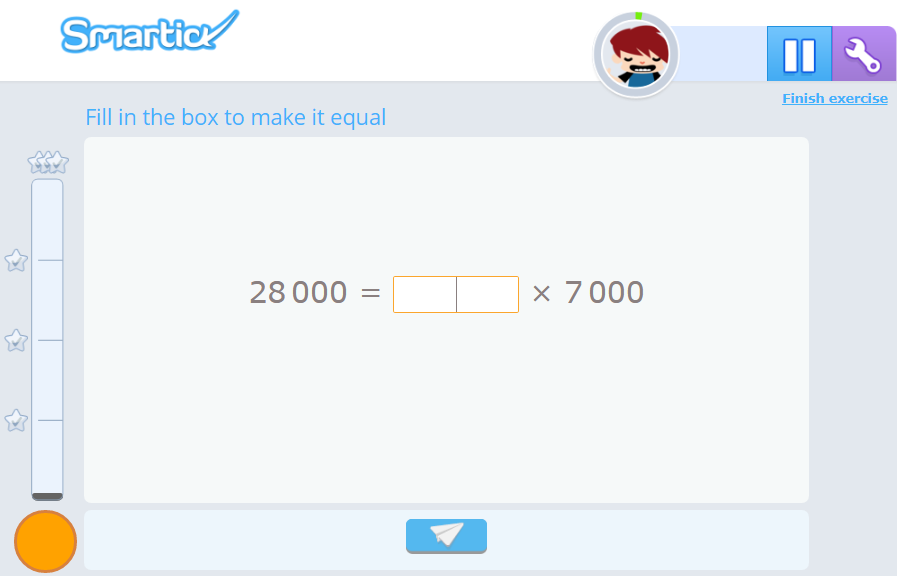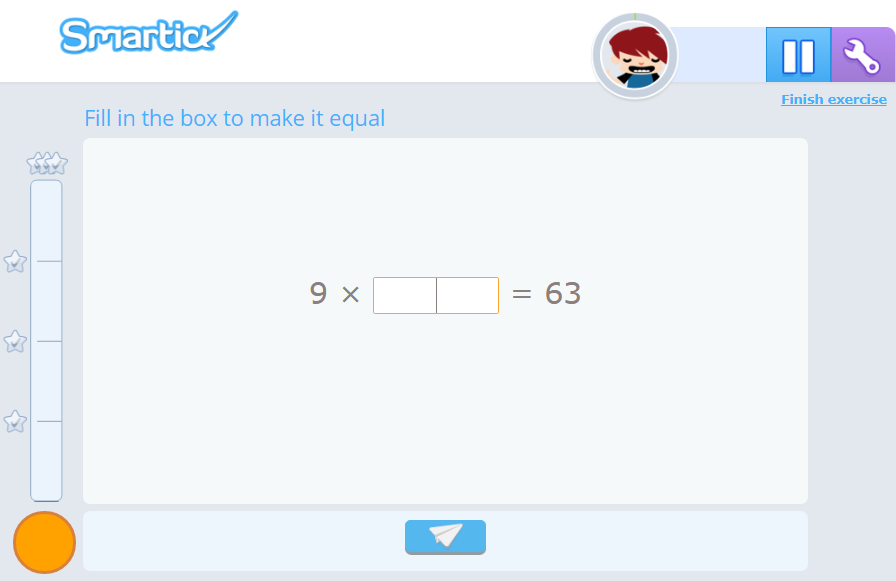Smartick is an advanced online program that teaches kids math and coding in only 15 min. a day

Dec15

# Completing Additions, Subtractions, Multiplications and Divisions

In today’s entry, we’re going to talk about some of the exercises that appear during the Smartick daily sessions. They may appear complex and convoluted at times, but they have great didactic potential.

They are exercises that require the student to complete a basic operation (addition, subtraction, multiplication, or division). These activities help the student understand different mathematical processes and develop strategies related to the resolution of operations. For example:

• Understanding the relation that exists between addition – subtraction, and between multiplication – division.
• Learning to break down numbers is essential for the student to develop their own strategies for solving basic operations.

That’s why, in Smartick, you’ll find these types of exercises reinforced by a great variety of word problems that cover all the semantic categories and exercises for the development of additive strategies.

We’re going to present some examples and explain the importance of each of them, the skills that they work on, and, of course, how to solve them. Let’s do it!These exercises develop the strategy of completing additions up to the next ten. In order words, it helps students think about the distance to the next ten on the number line. This helps us when we add, for example:

27+12

So, 27 is just 3 away from 30, so first we add 3 to get to 30, and then we add what's left, which is much easier. This gives us:

30+9


### Completing SubtractionsHere we find its opposite in subtraction. Both cases continue with exercises in completing additions and subtractions, reaching higher and lower non-consecutive tens.

### Completing MultiplicationsThe exercises in which the student is asked to complete multiplications (or divisions) work on the effect of units followed by zeros in a product or quotient, such as in the example above. On the other hand, as shown in the image below, the student works on reinforcement of their multiplication tables and the development of the understanding of the relationship that exists between multiplications and divisions.So, although an adult would solve these exercises by doing the inverse operation, a child will use other methods until they are able to understand such an abstract concept:

• Counting up to the next number (using their fingers, and it is positive that they do so).
• Making groups according to divisions or multiplications.
• Reviewing their time’s tables, number by number.

Now, if you want to keep practicing all these strategies and developing your mathematical competence, go to Smartick and discover a different, fun way to learn.